# How to Zip two or more Arrays in JavaScript - Complete GuideLast updated: Apr 16, 2023
6 min## #How to Zip two Arrays in JavaScript

To zip two arrays in JavaScript:

1. Use the `Array.map()` method to iterate over the first array.
2. On each iteration, return the current element and the corresponding element from the second array.
index.js
```Copied!```const arr1 = [1, 2, 3];

const arr2 = ['bobby', 'hadz', 'com'];

const result = arr1.map((element, index) => {
return [element, arr2[index]];
});

// 👇️ [ [ 1, 'bobby' ], [ 2, 'hadz' ], [ 3, 'com' ] ]
console.log(result);
``````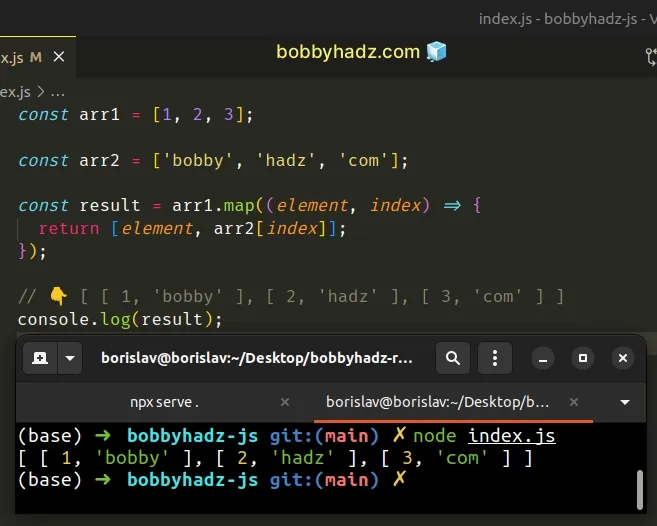The function we passed to the Array.map() method gets called with each element of the first array.

We also make use of the `index` parameter.

On each iteration, we return a new array that contains the current element of the first array and the corresponding element of the second array.

The result is a two-dimensional array that contains subarrays where the first element is taken from the first array and the second element is taken from the second array.

You can also shorten this a little by using an implicit return statement.

index.js
```Copied!```const arr1 = [1, 2, 3];

const arr2 = ['bobby', 'hadz', 'com'];

const result = arr1.map((element, index) => [
element,
arr2[index],
]);

// 👇️ [ [ 1, 'bobby' ], [ 2, 'hadz' ], [ 3, 'com' ] ]
console.log(result);
``````

This does the same as the Python zip function which iterates over several iterables in parallel and produces tuples with an item from each iterable.

main.py
```Copied!```list1 = [1, 2, 3]

result = list(zip(list1, list2))

# 👇️ [(1, 'bobby'), (2, 'hadz'), (3, 'com')]
print(result)
``````

## #Defining a reusable zip() function

If you have to zip two arrays often, define a reusable function.

index.js
```Copied!```function zip(arr1, arr2) {
return arr1.map((element, index) => {
return [element, arr2[index]];
});
}

const array1 = [1, 2, 3];
const array2 = ['bobby', 'hadz', 'com'];

const result = zip(array1, array2);

// 👇️ [ [ 1, 'bobby' ], [ 2, 'hadz' ], [ 3, 'com' ] ]
console.log(result);
``````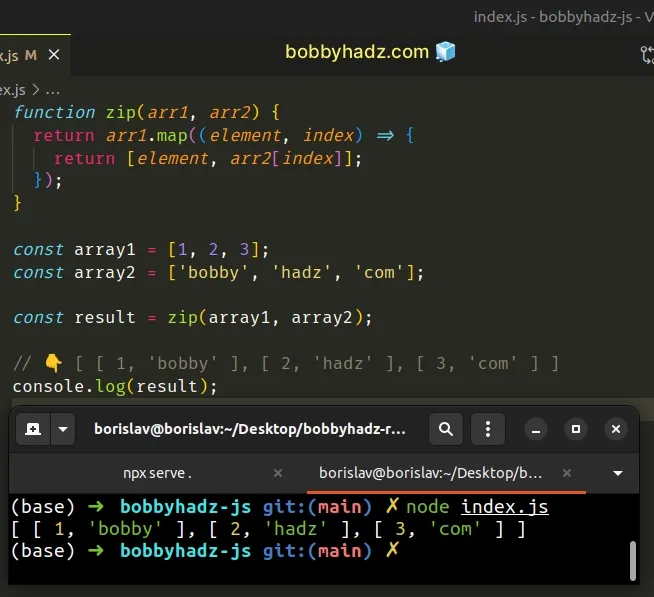The `zip()` function takes two arrays as parameters, zips the arrays and returns the result.

## #Zip two arrays of different lengths

When using this approach, you should consider the edge case where the arrays have a different length.

Here is an example where the first array is longer than the second array.

index.js
```Copied!```function zip(arr1, arr2) {
return arr1.map((element, index) => {
return [element, arr2[index]];
});
}

const array1 = [1, 2, 3, 4];
const array2 = ['bobby', 'hadz', 'com'];

const result = zip(array1, array2);

// 👇️ [ [ 1, 'bobby' ], [ 2, 'hadz' ],
//      [ 3, 'com' ], [ 4, undefined ] ]
console.log(result);
``````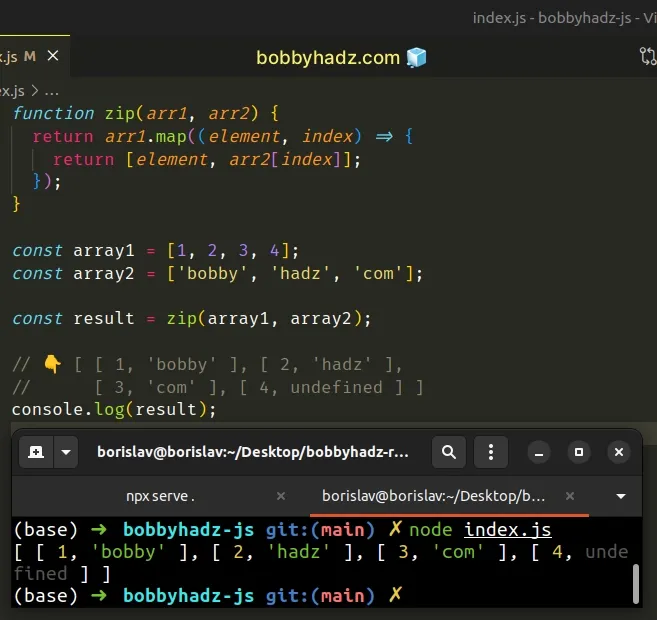Accessing the second array at an index that doesn't exist returns `undefined`, so the last subarray stores the values `4` and `undefined`.

However, if the first array has fewer elements than the second array, the remaining elements won't get added to the result.

index.js
```Copied!```function zip(arr1, arr2) {
return arr1.map((element, index) => {
return [element, arr2[index]];
});
}

const array1 = [1, 2, 3];
const array2 = ['bobby', 'hadz', 'com', 'abc'];

const result = zip(array1, array2);

// 👇️ [ [ 1, 'bobby' ], [ 2, 'hadz' ], [ 3, 'com' ] ]
console.log(result);
``````

The first array has 3 elements and the second array has 4.

We only iterate over the first array, so the fourth element of the second array is never added to the zipped array.

If you want to produce a consistent result, create a new array that has a length equal to the larger of the two arrays and iterate over it instead.

index.js
```Copied!```function zip(arr1, arr2) {
return Array(Math.max(arr1.length, arr2.length))
.fill()
.map((_, index) => [arr1[index], arr2[index]]);
}

const array1 = [1, 2, 3];
const array2 = ['bobby', 'hadz', 'com', 'abc'];

const result = zip(array1, array2);

// 👇️ [ [ 1, 'bobby' ], [ 2, 'hadz' ],
//      [ 3, 'com' ], [ undefined, 'abc' ] ]
console.log(result);
``````

We used the Math.max() method to get the length of the larger array and used the `map()` method to iterate over the new array.

On each iteration, we access the arrays at the current index and return a new array containing the two values.

Now all array elements are guaranteed to be contained in the zipped array regardless if the first array is longer than the second or vice versa.

## #Zip multiple arrays in JavaScript

If you need to zip multiple arrays in JavaScript:

1. Use the `Math.max()` method to get the length of the longest array.
2. Use the `Array()` constructor to create an array of the given length.
3. Use the `map()` method to iterate over the new array.
4. On each iteration, access the subarray at the current index and return the result.
index.js
```Copied!```function zip(...arrays) {
return Array(Math.max(...arrays.map(arr => arr.length)))
.fill()
.map((_, index) => arrays.map(arr => arr[index]));
}

const array1 = [1, 2, 3];
const array2 = ['bobby', 'hadz', 'com'];
const array3 = ['a', 'b', 'c'];

const result = zip(array1, array2, array3);

// [ [ 1, 'bobby', 'a' ],
//   [ 2, 'hadz', 'b' ],
//   [ 3, 'com', 'c' ] ]
console.log(result);
``````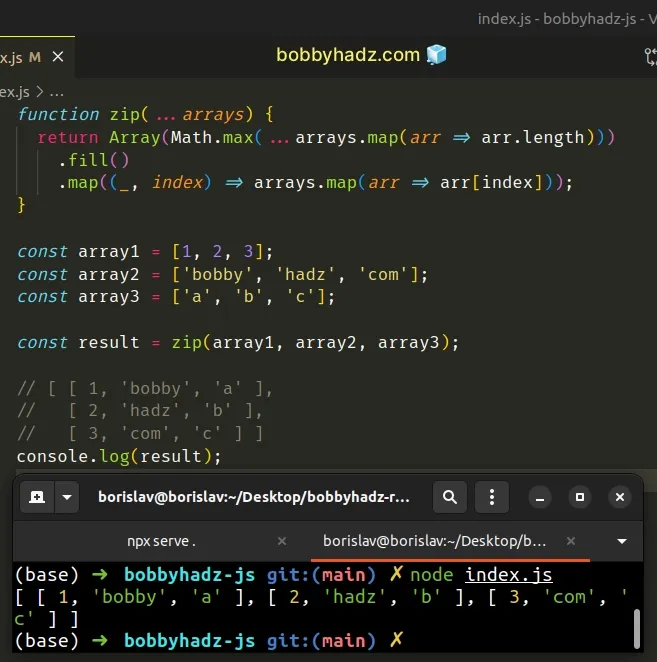Notice that we used the `...gather` operator in the function's definition.

The operator gathers the supplied arrays into a new array.

Here is an example that demonstrates how this works.

index.js
```Copied!```function zip(...arrays) {
// [ [ 1, 2, 3 ],
// [ 'bobby', 'hadz', 'com' ],
// [ 'a', 'b', 'c' ] ]
console.log(arrays);
}

const array1 = [1, 2, 3];
const array2 = ['bobby', 'hadz', 'com'];
const array3 = ['a', 'b', 'c'];

const result = zip(array1, array2, array3);
``````

Notice that the `...` operator simply gathers the arrays into a new array.

The next step is to use the `Math.max()` method to get the length of the longest array.

index.js
```Copied!```return Array(Math.max(...arrays.map(arr => arr.length)))
.fill()
.map((_, index) => arrays.map(arr => arr[index]));
``````

Once we create a new array of the given length, we use the `.map()` method to iterate over the array.

On each iteration, we use the `map()` method to iterate over the two-dimensional array that we previously gathered using the `...` syntax and return the subarray element at the current index.

This implementation of the `zip()` function can be used to zip as many arrays as necessary.

index.js
```Copied!```function zip(...arrays) {
return Array(Math.max(...arrays.map(arr => arr.length)))
.fill()
.map((_, index) => arrays.map(arr => arr[index]));
}

const array1 = [1, 2, 3];
const array2 = ['bobby', 'hadz', 'com'];
const array3 = ['a', 'b', 'c'];

const result = zip(array1, array2, array3);

// [ [ 1, 'bobby', 'a' ],
//   [ 2, 'hadz', 'b' ],
//   [ 3, 'com', 'c' ] ]
console.log(result);
``````

## #Zip two arrays in JavaScript using the `reduce()` method

You can also use the Array.reduce method to zip two arrays in JS.

index.js
```Copied!```function zip(arr1, arr2) {
return arr1.reduce((accumulator, curr, index) => {
return [...accumulator, [curr, arr2[index]]];
}, []);
}

const array1 = [1, 2, 3];
const array2 = ['bobby', 'hadz', 'com'];

const result = zip(array1, array2);

// 👇️ [ [ 1, 'bobby' ], [ 2, 'hadz' ], [ 3, 'com' ] ]
console.log(result);
``````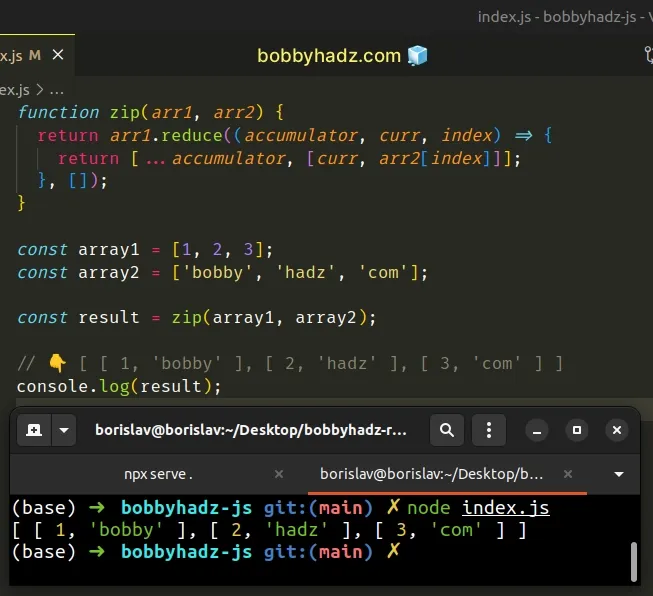We initialized the `accumulator` variable to an empty array because that's what we passed as the second argument to the `reduce()` method.

On each iteration, we use the spread syntax (...) to unpack the accumulator array into a new array and add the current elements from the two arrays.

## #Zip two arrays in JavaScript using the `forEach()` method

Alternatively, you can use the Array.forEach method.

index.js
```Copied!```function zip(arr1, arr2) {
const zipped = [];

arr1.forEach((element, index) => {
zipped.push([element, arr2[index]]);
});

return zipped;
}

const array1 = [1, 2, 3];
const array2 = ['bobby', 'hadz', 'com'];

const result = zip(array1, array2);

// 👇️ [ [ 1, 'bobby' ], [ 2, 'hadz' ], [ 3, 'com' ] ]
console.log(result);
``````

The function we passed to the `Array.forEach()` method gets called with each element and index from the array.

On each iteration, push a new array into the `zipped` array.

The array contains the current element and the corresponding element from the second array.

## #Zip two arrays in JavaScript using a `for` loop

You can also use a simple `for` loop to zip two arrays.

index.js
```Copied!```function zip(arr1, arr2) {
const zipped = [];

for (let index = 0; index < arr1.length; index++) {
zipped.push([arr1[index], arr2[index]]);
}

return zipped;
}

const array1 = [1, 2, 3];
const array2 = ['bobby', 'hadz', 'com'];

const result = zip(array1, array2);

// 👇️ [ [ 1, 'bobby' ], [ 2, 'hadz' ], [ 3, 'com' ] ]
console.log(result);
``````

The syntax for a basic `for` loop is quite verbose compared to the `Array.forEach()` method, however, the concept is the same.

We iterate over the first array and push a new array containing the current elements from the two arrays into the `zipped` array.

Which approach you pick is a matter of personal preference. I'd use the `Array.map()` method as I find it quite direct and intuitive.